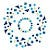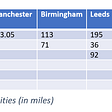# Hyper-Parameter Tuning!!

But, Before moving on Let’s understand how is it a important task in any machine learning Project.

# Difference between Parameters and Hyperparameters

Now that we are clear with the difference between model parameters and hyperparameters. Let’s take a look at ways to find the optimal hyperparameters values.

# Hyperparameter Tuning/Optimization

Building a baseline Model using RandomForest using the Titanic data.

`import pandas as pdfrom sklearn.model_selection import train_test_splitfrom sklearn.ensemble import RandomForestClassifierfrom sklearn.metrics import accuracy_score,f1_score,precision_score,recall_scoredata = pd.read_csv("/content/train (1).csv")`
`data.isnull().sum()`
`data.Age.fillna(29,inplace=True)data.drop(['PassengerId','Cabin','Ticket','Name'],axis = 1,inplace= True)data.dropna(inplace=True)`
`data = pd.get_dummies(data,drop_first=True)data.Pclass = data['Pclass'].astype(object)`
`x = data.drop('Survived',axis = 1)y = data['Survived']x_train,x_test,y_train,y_test = train_test_split(x,y,test_size = 0.1,random_state = 123)`
`def evaluate_model(x_test,y_test,model):    pred = model.predict(x_test)    print("Accuracy is : {}".format(accuracy_score(y_test,pred)))    print("------------------------------------------")     print("F1-Score is : {}".format(f1_score(y_test,pred)))    print("------------------------------------------")    print("Precision is : {}".format(precision_score(y_test,pred)))    print("------------------------------------------")    print("Recall is : {}".format(recall_score(y_test,pred)))`
`rf = RandomForestClassifier().fit(x_train,y_train)evaluate_model(x_test,y_test,rf)`

Our baseline model is performing pretty well let’s see if we can improve it’s performance by using different hyperparameter tuning methods.

# Different Methods of Hyperparameter Tuning are:

## → GridSearch:

`from pprint import pprintrf = RandomForestClassifier()# Look at parameters used by our current forestprint('Parameters currently in use:\n')pprint(rf.get_params())`
`# Number of trees in random forestn_estimators = [int(x) for x in np.linspace(start = 200, stop = 1500, num = 4)]# Number of features to consider at every splitmax_features = ['auto', 'sqrt']# Maximum number of levels in treemax_depth = [int(x) for x in np.linspace(10, 80, num = 4)]# Minimum number of samples required to split a nodemin_samples_split = [2, 5, 10]# Minimum number of samples required at each leaf nodemin_samples_leaf = [1, 2, 4]# Method of selecting samples for training each treebootstrap = [True, False]grid_para = {'n_estimators': n_estimators,               'max_features': max_features,               'max_depth': max_depth,               'min_samples_split': min_samples_split,               'min_samples_leaf': min_samples_leaf,               'bootstrap': bootstrap}pprint(grid_para)#print our grid of hyperparameter values`
`from sklearn.model_selection import GridSearchCVgrid_search = GridSearchCV(estimator = rf, param_grid = grid_para,                           cv = 3, n_jobs = -1, verbose = 1)# Fit the random search modelgrid_search.fit(x_train,y_train)grid_search.best_params_ #outputs the set of best hyperparameter                                                        values.`

Notice, we are getting around 1728 fits, this is because we are cross validating our model 3 times.
So, 576 * 3 = 1728

`evaluate_model(x_test,y_test,grid_search)`

It’s clearly seen that each one of our evaluation metrics has increased drastically.
Now, Let’s do the same for the other method.

## → RandomSearch:

`from pprint import pprintrf = RandomForestClassifier()# Look at parameters used by our current forestprint('Parameters currently in use:\n')pprint(rf.get_params())`
`# Number of trees in random forestn_estimators = [int(x) for x in np.linspace(start = 200, stop = 1500, num = 4)]# Number of features to consider at every splitmax_features = ['auto', 'sqrt']# Maximum number of levels in treemax_depth = [int(x) for x in np.linspace(10, 80, num = 4)]# Minimum number of samples required to split a nodemin_samples_split = [2, 5, 10]# Minimum number of samples required at each leaf nodemin_samples_leaf = [1, 2, 4]# Method of selecting samples for training each treebootstrap = [True, False]random_grid = {'n_estimators': n_estimators,               'max_features': max_features,               'max_depth': max_depth,               'min_samples_split': min_samples_split,               'min_samples_leaf': min_samples_leaf,               'bootstrap': bootstrap}pprint(random_grid)#print our grid of hyperparameter values`
`from sklearn.model_selection import RandomizedSearchCVrf_random = RandomizedSearchCV(estimator = rf, param_distributions = random_grid, n_iter = 60, cv = 3, verbose=1, random_state=42, n_jobs = -1)# Fit the random search modelrf_random.fit(x_train,y_train)rf_random.best_params_ #outputs the set of best hyperparameter                                                        values.`

Our overall accuracy has increased, However, The increase in accuracy is due to drastic increase in Precision, but, Our recall score has reduced.

# Reference:

--

--

--

## More from INSAID

One of India’s leading institutions providing world-class Data Science & AI programs for working professionals with a mission to groom Data leaders of tomorrow!

Love podcasts or audiobooks? Learn on the go with our new app.

## Human Face, emotion and race detection with python## “Quantum algorithm” Science-Research, September 2021, Week 4 — summary from Astrophysics Data…## SEE YOU IN CVPR 2022## Ecological Protection through Object Detection at Renewable Wind Farms## Computer Vision 101: Working with Color Images in Python## Intro to ML Ops : Tensorflow Extended (TFX)## Bias-Variance tradeoff in Machine Learning models: A practical example## INSAID

One of India’s leading institutions providing world-class Data Science & AI programs for working professionals with a mission to groom Data leaders of tomorrow!

## Kaggle — Black Friday dataset using XGBoost algorithm + Feature Engineering## Hierarchical Clustering using an example## Model Building## Fake Job prediction with Machine Learning